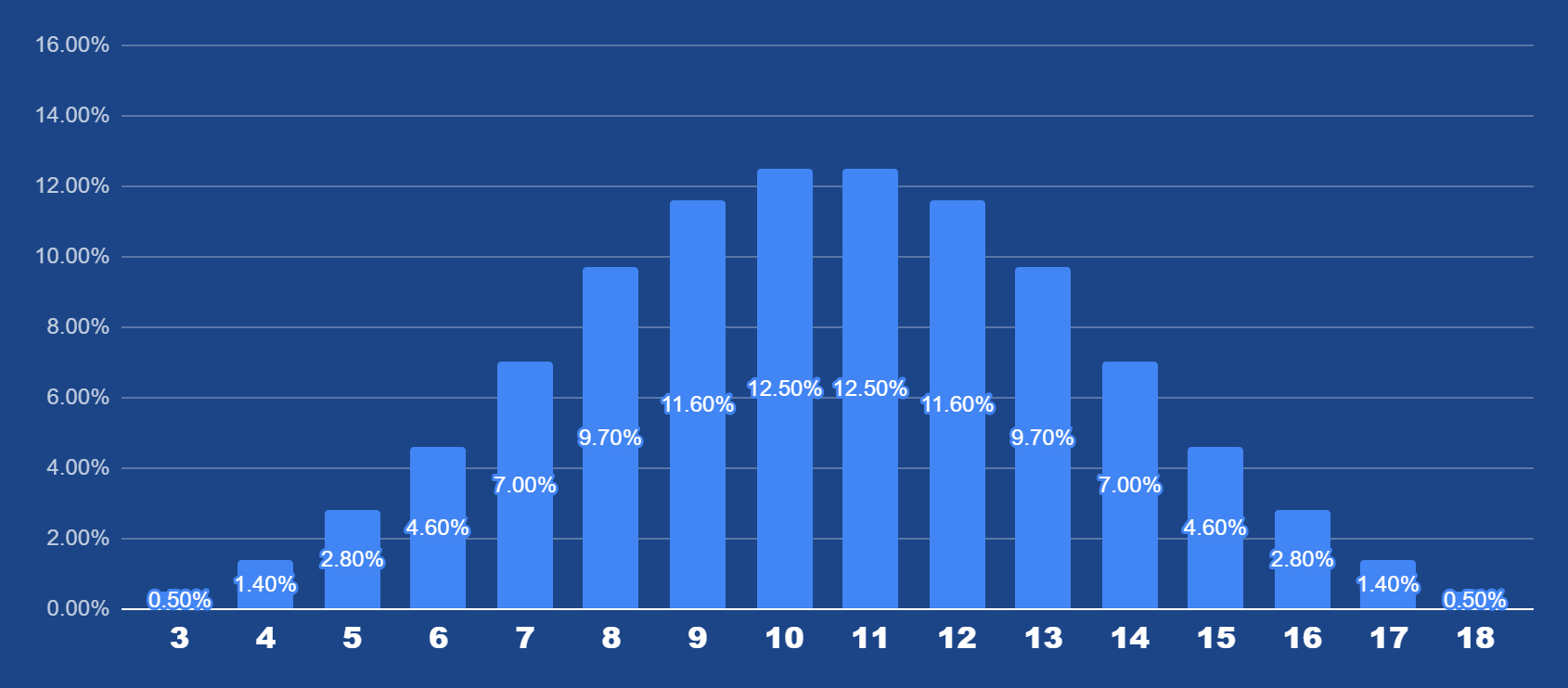Lightning Dice from Evolution Gaming is a casino game show with a 96.21% RTP. The game show host or hostess drops three 6-sided dice into a twisting and turning transparent "Lightning Tower" that tumbles towards the bottom to reveal the outcome.

The goal is to guess what number between 3 and 18 will be the result. Before the host drops the dice into the tower, a lightning bolt will randomly strike two or three possible outcomes and reveal a random multiplier of up to 1.000x.

## Lightning Dice Rules

• Bets accepted are from €1 to €2.000 per round.
• There are 16 available bets, each corresponding to an outcome of the dice tumble (from 3 to 18).
• Payouts vary per outcome, from 5x your bet to 150x.
• You place your bet(s) on the numbered outcome of the tumble, from 3 to 18.
• A lightning bolt will randomly strike two or three numbers before the round begins.

## Lightning Dice Payouts

Sum of all dice : x(bet amount) payout.

 Number Multiplier 3 150x 4 50x 5 25x 6 15x 7 10x 8 7x 9 6x 10 5x 11 5x 12 6x 13 7x 14 10x 15 15x 16 25x 17 50x 18 150x

### Lightning Dice Odds• Probability of a sum of 3: 1/216 = 0.5%
• Probability of a sum of 4: 3/216 = 1.4%
• Probability of a sum of 5: 6/216 = 2.8%
• Probability of a sum of 6: 10/216 = 4.6%
• Probability of a sum of 7: 15/216 = 7.0%
• Probability of a sum of 8: 21/216 = 9.7%
• Probability of a sum of 9: 25/216 = 11.6%
• Probability of a sum of 10: 27/216 = 12.5%
• Probability of a sum of 11: 27/216 = 12.5%
• Probability of a sum of 12: 25/216 = 11.6%
• Probability of a sum of 13: 21/216 = 9.7%
• Probability of a sum of 14: 15/216 = 7.0%
• Probability of a sum of 15: 10/216 = 4.6%
• Probability of a sum of 16: 6/216 = 2.8%
• Probability of a sum of 17: 3/216 = 1.4%
• Probability of a sum of 18: 1/216 = 0.5%

As you can see above, the probability of 3 and 18 rolling is the least, at only 0.5%. Hence, the massive 150x payout. If a Lightning for 1000x strikes on 3 or 18, you're on your way to buying a Villa in Las Vegas!

The most probable outcomes are the middle spots: 10 and 11, followed by 9 and 13, and so on.